# Beginners guide to Amiga E

I have now written about two intepretating languages in the Amiga echosystem, Amiga Basic and Arexx. It is time for write about compiling languages, because if you're writing LOB applications or even games, you would want to compile the code down to machine code, or in some cases write the assembler directly.

I assume that a lot of programming for the Amiga was done in C, and compiled with Lattice C or SAS/C, but writing about C programming is boring as hell. Instead I want to shine some light of another very popular programming language on the Amiga, nameley the Amiga E programming language.

Amiga E was created by Wouter van Oortmerssen in 1991 and was released as shareware on the Amiga in 1993. In 1997 it was open sourced and free to use. The whole compiler was written by Wouter himself in one 400kb large M68000 assembler file. I think we can conclude that Wouter van Oortmerssen is a genious, and slightly mental.

## Hello World

Our first program in Amiga E will be a very simple one. I will once again use the memacs editor to write my programs.

``````PROC main()
WriteF('Hello World\n')
ENDPROC
``````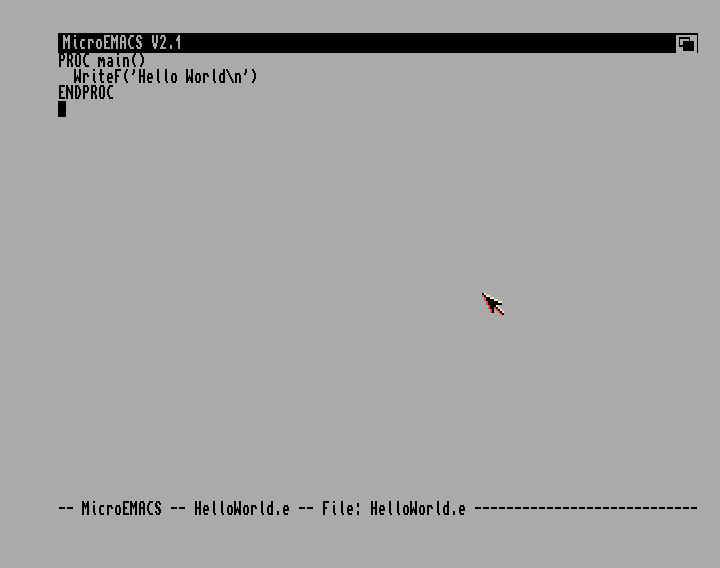The Amiga E language is supposed to be inspired by C and C++, but this seems more like Pascal to me.

We compile the program from CLI to get a runnable binary.

``````ec HelloWorld.e
``````

I always find it interesting to see the size of a program after compilation. How many instructions my code turned into. This simple example turned into a binary of 672 bytes. I assume there is some padding in there, and the program would be smaller if I wrote it in M68000 assembler, but it is at least <1kb which is totally acceptable.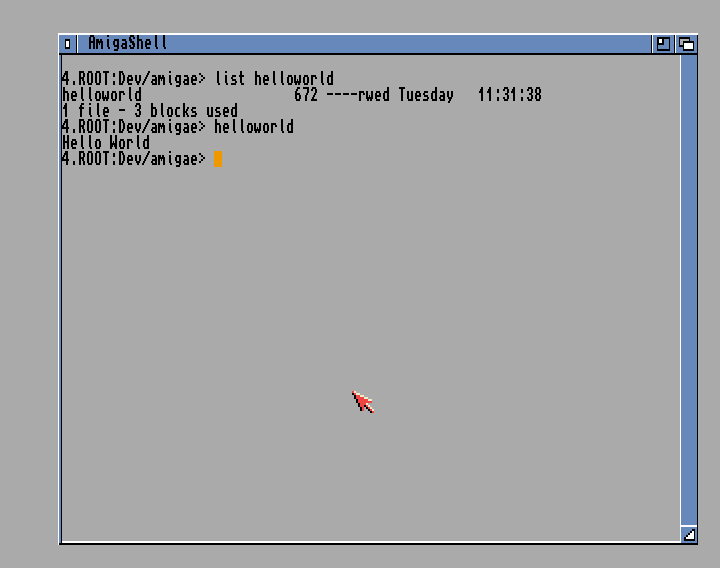## Variables

Variables in Amiga E are losely typed, but as with Amiga Basic you need to define them before using them.

``````PROC main()
DEF width, height, area
width := 12
height := 4
area := width * height

WriteF('With the width \d and the height \d', width, height)
WriteF(' you have the area \d\n', area)
ENDPROC
``````

I sense several influences in this code.

• Variables are declared as in Amiga Basic, just a name but no type
• Variables are assigned as in Pascal
• WriteF reminds me of printf from C, but there you use %d instead of \d to convert decimals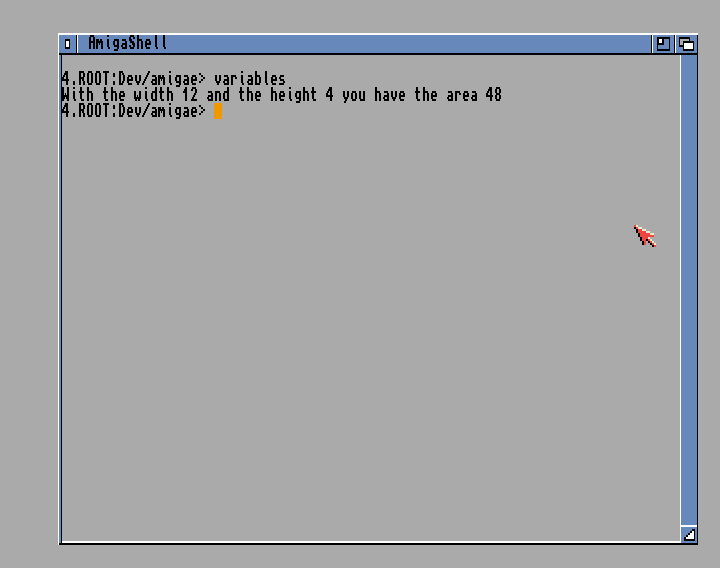Dealing with floating point numbers in Amiga E is a bit tricky as the language doesn't really have it as a type, but using LONG to store floats. This means that when you're handling floats you are handling longs, meaning you need to specify every time you need a float operation instead of a long operation. This is done by a special casting technique with the `!` operator.

If you prepend your field with the `!` operator it will convert the long value to a float. If you postpend the field you will convert to an integer. Here is an example on how to calculate the area of a circle in Amiga E.

``````CONST PI=1078523331

PROC main()

WriteF(' we have the area \s\n', RealF(output, area, 2))
ENDPROC
``````

There is some really crazy things going on in this code.

• `CONST PI=1078523331` doesn't look like PI but it is. As float is not a first level type in Amiga E we can't create constants that are float. We can create a constant LONG that will become 3.14 when it is converted to float. That is what's happening here.

• `output:STRING` defines a string variable of 20 characters that we will use to output float numbers with WriteF, because WriteF can only handle LONG.

• `!radius*radius*(!PI)` first convert the LONG PI number to float, then multiply it by the float converted radius and it will become the area.

• `RealF(output, radius, 1)` converts the LONG in radius to a float in a string that can be outputted to WriteF as a \s (string).

As strangely as this might look, it actually works.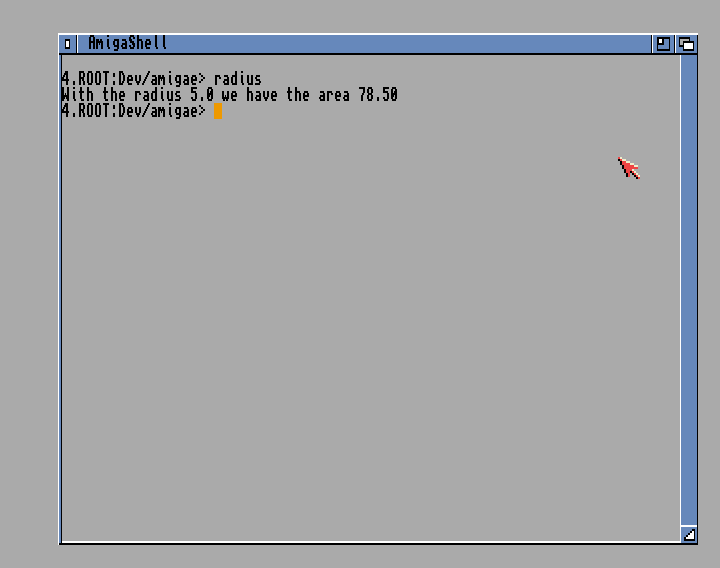Getting input from the user on the command line is pretty straight forward in Amiga E.

``````PROC main()
DEF name:STRING, size:STRING

WriteF('What is your shoe size? ')

WriteF('Hello \s, your shoe size is \s\n', name, size)
ENDPROC
``````
• `ReadStr` is a function that you use to read from a file, and the file in question is stdin (the console input). It will read until it reaches EOF, which will happen when the user presses ENTER.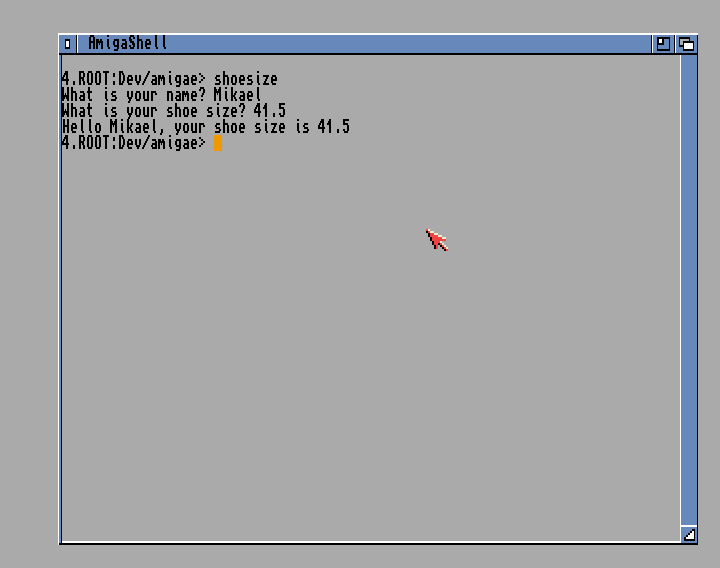## Control structures

It is time to start looping in Amiga E. Here is a small game where you should guess what number the computer is thinking of.

``````CONST MAX=999

PROC main()
DEF number, guess, guess_str:STRING, guesses

WriteF('The number is between 1-1000.\n')

/* Initialize values */
number := Rnd(MAX - 1) + 1
guess := -1
guesses := 0

WHILE guess <> number
WriteF('Guess the number: ')
guess := Val(guess_str)

IF guess > number
WriteF('Your guess "\d" was too high\n', guess)
ELSEIF guess < number
WriteF('Your guess "\d" was too low\n', guess)
ENDIF

guesses := guesses + 1
ENDWHILE

WriteF('You found the number in \d tries.', guesses)
ENDPROC
``````

This code is pretty straight forward.

• `Rnd(n)` will return a number in the interval 0-(n-1) so if we want a number in the 1-999 interval we need to do `Rnd(998) + 1)`

• `ReadStr(stdin, guess_str)` puts the readed value in guessstr. We then need to parse that value with `Val(guessstr)` in order to get the numeric value in guess.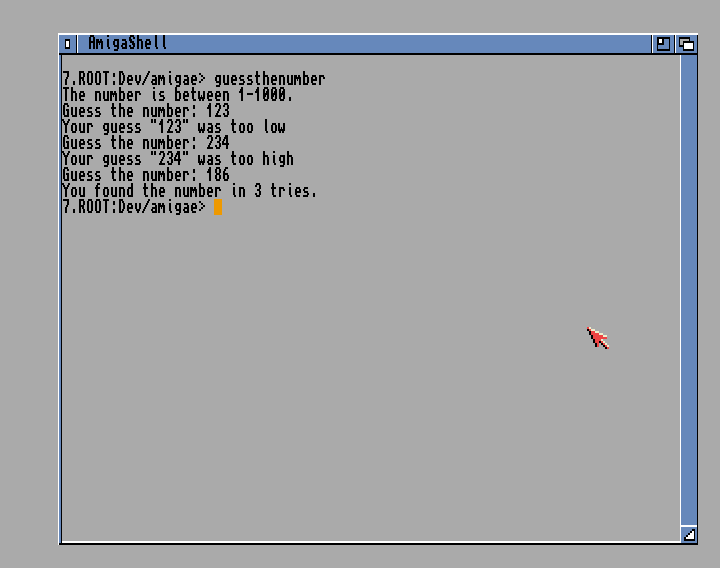## For-loops

For-loops in Amiga E is pretty similiar to Pascal where you need to define an identifier for the iterator and you can loop to an expression that is calculated once.

Here is an example that will type out a pyramid of stars to the screen.

``````PROC main()
DEF i, j, height, height_str:STRING

WriteF('Height of pyramid: ')
height := Val(height_str)

FOR i:=1 TO height

/* Empty spaces before building blocks */
FOR j:=1 TO (height - i)
WriteF(' ')
ENDFOR

/* Building blocks */
FOR j:=1 TO (i + i + 1)
WriteF('*')
ENDFOR

WriteF('\n')
ENDFOR
ENDPROC
``````

This algorithm is very inefficient as we're writing every character one by one, but it shows very well how For-loops works in Amiga E.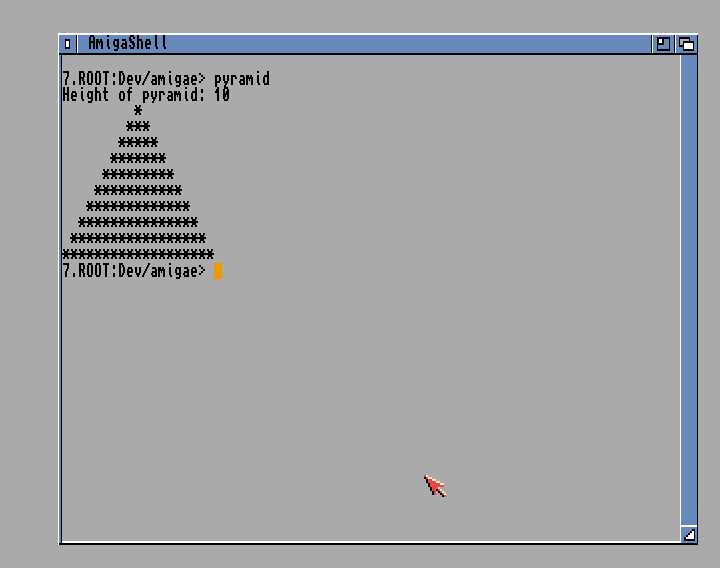We can also specify how the iterator should be incremented, and this allow us to count down.

``````PROC main()
DEF i, n, n_str:STRING, result

WriteF('Calculate the factorial of: ')
n := Val(n_str)

result := 1
FOR i:=n TO 1 STEP -1
result := result * i
ENDFOR

WriteF('\d! = \d\n', n, result)
ENDPROC
``````
• `FOR i:=n TO 1 STEP -1` is the magic that makes the FOR-loop to count downwards instead of up.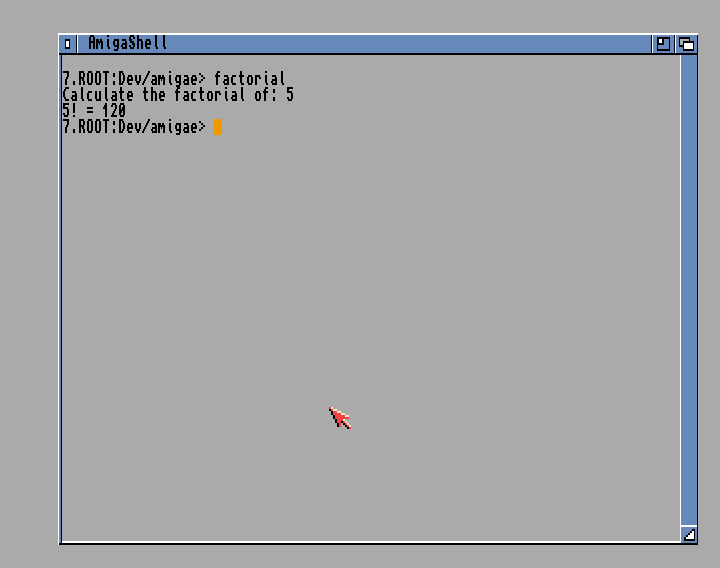## Select-Case

When IF-ELSEIF-ELSE statements become too complicated, Amiga E has a structure called SELECT-CASE that you can use to tidy things up. This is like most CASE-statements in other languages, but a bit more powerful.

We can use this to build a menu.

``````PROC main()
DEF input, input_str:STRING

input := 0
WriteF('Welcome, please select any of the following\n')
WriteF('1. Vegetables\n')
WriteF('2. Fruit\n')
WriteF('3. Gardening tools\n')
WriteF('4. Electronic equipment\n')
WriteF('or 5 to quit\n\n')

WHILE input<>5
WriteF('> ')
input := Val(input_str)

SELECT 6 OF input
CASE 1 TO 2
WriteF('You selected food\n')
CASE 3, 4
WriteF('You selected a tool\n')
CASE 5
WriteF('Thank you, and welcome back!\n')
DEFAULT
WriteF('Unknown selection\n')
ENDSELECT
ENDWHILE
ENDPROC
``````

Here we create a selection between 0-6 and we select a case depending on input. Input could be any kind of expression that is evaluated when the program comes to that point. The default clause is run if there is no matching case.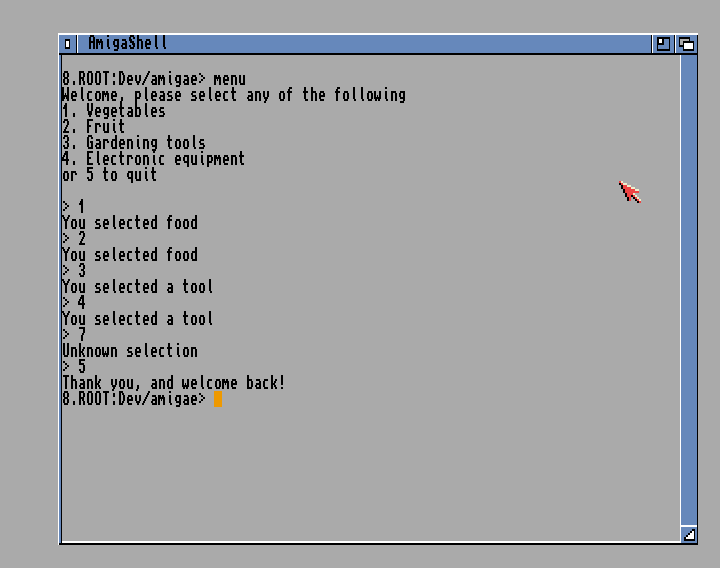## Strings

When we define a string it is of a fixed length. We have no way to define a string of variable string that is common in most modern programming languages.

Here is a string manipulations example, doing ceasar ciphers

``````CONST ALPHABET=25

PROC main()
DEF data:PTR TO CHAR, result:PTR TO CHAR
DEF encKey, encKey_str:STRING, i
DEF enchChar:STRING

WriteF('Enter the encryption key (number): ')
encKey := Val(encKey_str)

WriteF('Enter the phrase to encrypt (capital letters): ')

result := String(EstrLen(data))

FOR i := 0 TO (EstrLen(data) - 1)
encChar := Mod(data[i] - 65 + encKey, ALPHABET) + 65
result[i] := encChar
ENDFOR

WriteF('Encrypted phrase: \s\n', result)
ENDPROC
``````

There are two types of strings in Amiga E. There is the STRING type and the e-string. The difference is how they are defined. The STRING type has a fixed size set when the variable is defined. The size of the e-string is set when the variable is initialized. So a STRING will always have the same size. The size of the e-string is defined at runtime.

On a more technical standpoint, a STRING is an array of chars, where an e-string is a linked list of chars. All API functions that accept STRING will also accept e-string but not the other way around.

• `result:PTR TO CHAR` defines a new e-string. The result variable is a pointer to the first cell of the CHAR LIST.

• `result := String(EstrLen(data))` initializes the e-string with the same size as data.

You can index into a string in Amiga E just as with most other languages and both set and get individual chars.## Arrays

Arrays are a common type in most programming languages. We have been using arrays in the form of STRING which is an array of CHARS.

Here we will use an array to calculate the fibonacci sequence.

``````CONST MAX=10

PROC main()
DEF i, numbers[MAX]:ARRAY

numbers := 1
numbers := 2

/* Calculate fibonacci sequence */
FOR i:=2 TO (MAX - 1)
numbers[i] := numbers[i - 2] + numbers[i - 1]
ENDFOR

/* Print the sequence */
FOR i:=0 TO (MAX - 1)
WriteF('\d ', numbers[i])
ENDFOR

WriteF('\n')
ENDPROC
``````

If we like here define an array without specifying the type, it will default to char. The index of the array is zero-based and we can index into the array as in most programming languages.

We can also define an `ARRAY OF INT` or `ARRAY OF OBJ` if we want an array of custom objects.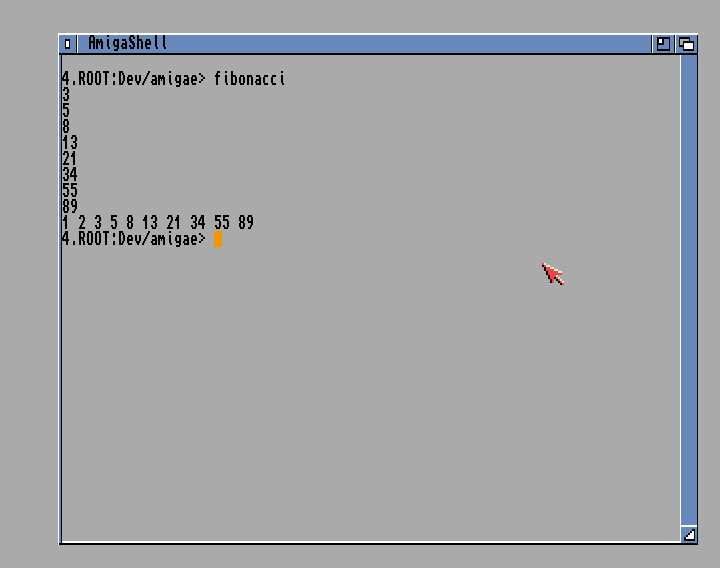## Procedures

Our program execution always start at the `main()` procedure. We can add other procedures to our program and execute those.

``````CONST WIDTH=31

PROC title()
WriteF('*** AMIGA E WILL LIVE AGAIN ***\n')
ENDPROC

PROC main()
separator()
title()
separator()
ENDPROC

PROC separator()
DEF i
FOR i:=1 TO WIDTH
WriteF('*')
ENDFOR
WriteF('\n')
ENDPROC
``````

You write procedures just as you write the main procedure. One noteable thing is that the procedure that you're calling doesn't have to before or after the callee. The order of the procedures doesn't matter.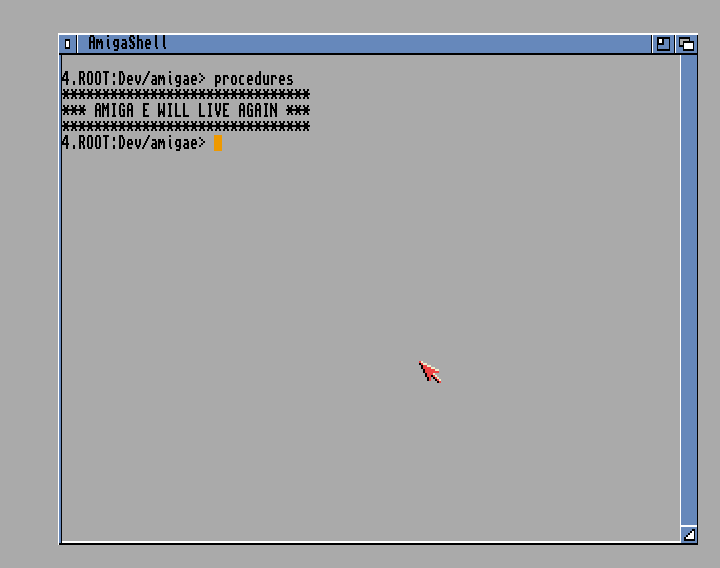Let's try another example with procedures. You can define variables outside procedures to make them global, and you can send values to procedures as arguments.

``````CONST MAX=20
DEF numbers[MAX]:ARRAY OF INT

/* Randomize numbers in array */
PROC randomize()
DEF i
FOR i:=0 TO (MAX - 1)
numbers[i] := Rnd(100)
ENDFOR
ENDPROC

/* Swap two values in the array */
PROC swap(x, y)
DEF temp
temp := numbers[x]
numbers[x] := numbers[y]
numbers[y] := temp
ENDPROC

PROC sort()
DEF i, j
FOR i:=0 TO (MAX - 2)
FOR j:=i+1 TO (MAX - 1)
IF numbers[i] > numbers[j]
swap(i, j)
ENDIF
ENDFOR
ENDFOR
ENDPROC

PROC print()
DEF i
FOR i:=0 TO (MAX - 1)
WriteF('\d ', numbers[i])
ENDFOR
WriteF('\n')
ENDPROC

PROC main()
randomize()
WriteF('An array of random numbers\n')
print()
WriteF('Sorting the array\n')
sort()
print()
ENDPROC
``````

There is not much happening here apart from sending values to the swap procedure and using numbers variable as a global array. This is starting to look like a real program and not just some sample code.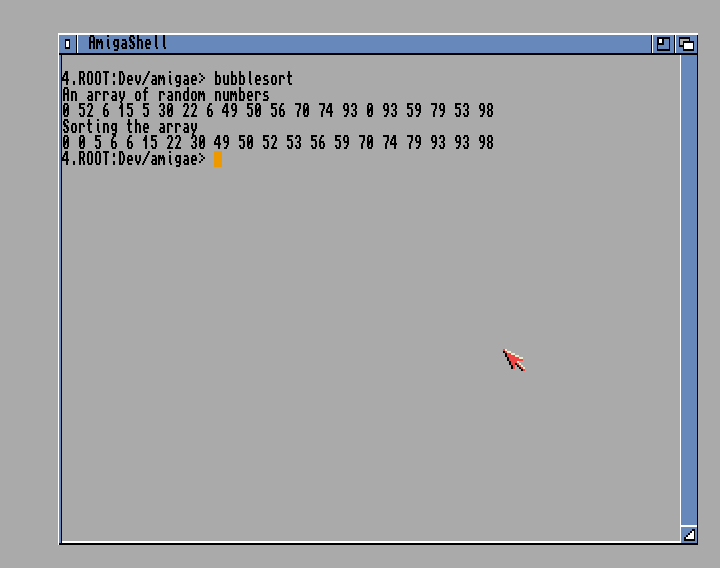## Functions and recursion

The only difference between a procedure and a function in Amiga E is that functions return values. Actually functions in Amiga E can return up to three values. Only the first value will be handled in expressions. In assignment you can get all the values.

``````x, y, z := get3DPoint()
``````

The main feature of functions is of course recursion, here demonstrated in a binary search algorithm.

``````PROC create()
DEF list:PTR TO CHAR
list:=[27, 29, 38, 42, 43, 47, 54, 59, 60, 62]:CHAR
ENDPROC list

PROC print(list)
DEF i
FOR i:=0 TO 9
WriteF('\d ', list[i])
ENDFOR
WriteF('\n')
ENDPROC

PROC exists(min, max, search, list)
DEF middle, found

IF (search = list[min]) OR (search = list[max])
found := TRUE

ELSEIF (max - min) < 2
found := FALSE

ELSE
middle := min + ((max - min) / 2)
IF (search >= list[middle])
found := exists(middle, max, search, list)

ELSE
found := exists(min, middle - 1, search, list)

ENDIF
ENDIF
ENDPROC found

PROC main()
DEF input_str:STRING, input, list

list := create()
print(list)

WriteF('Test if number is in vector: ')
input := Val(input_str)

IF exists(0, 9, input, list)
WriteF('The number \d exists\n', input)
ELSE
WriteF('The number \d didn\at exist\n', input)
ENDIF
ENDPROC
``````

Here I write a function create() that is responsible for creating a list of numbers. A list in Amiga E is not much different from an array, other than it is initialized in runtime so the size can be dynamic. This is called an e-list and works exactly the same way as an e-string.

The `ENDPROC` takes the argument of what is returned from the function. This way we might write a recursive function.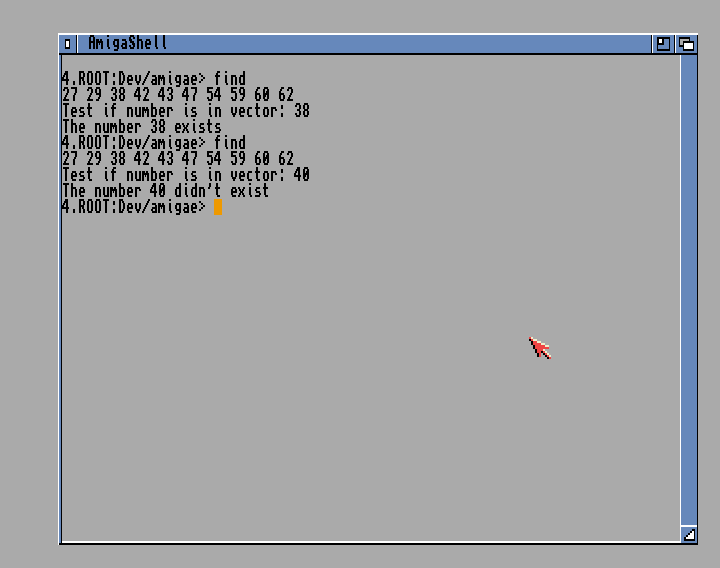## Summary

I have been going through the basics of Amiga E, and there are a few aspects that I haven't even touched. I have not showed you Enumerations, Sets, Object Oriented Programming or Linked Lists. There are more cool things like Unification, Quoted Expression and writing Assembly Statements directly in Amiga E source.

You can write your own modules and use the built in Amiga System Modules to create user interfaces.

I was surprised by Amiga E. It was not really what I expected it to be. It is a very close relative to Pascal, but where Pascal is a complete abstraction of what lies underneath I feel that Amiga E is more like syntactic sugar than a complete abstraction. The assembly layer is leaking through in stuff like, everything is a LONG variable, and indexing a list is depending on what size of the type inside the list.

For a language created by one person, it is still great and I think a much needed improvement over C/C++. The compiler is sometimes a bit quirky but that is expected. I think Amiga E is an awesome language and it could be called a modern programming language even though it is officially dead.